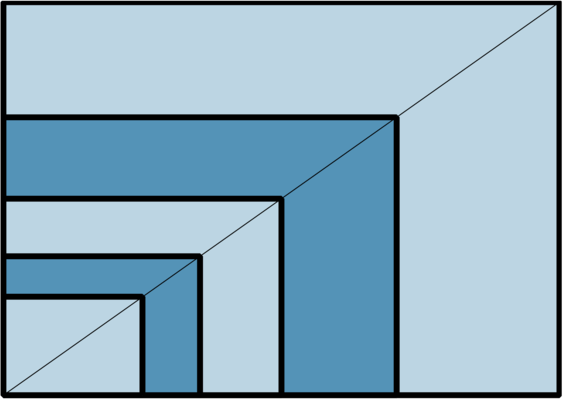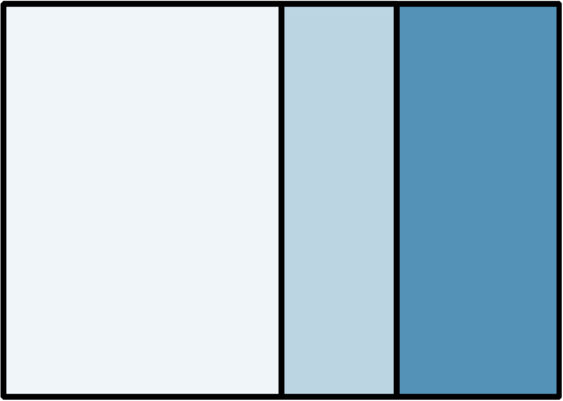# 2. Why does “A” paper work?

The problem from the first part of this series was to prove the method of folding the diagonal of a rectangle. Figure 2-1 shows the result of the first step. The diagonal of the rectangle is the line that goes through two opposite corners, and therefor the centre. Folding together the rectangle’s other opposite corners makes an isosceles triangle – the two equal sides are shown in heavy orange line. When we fold the pinch marks together (shown with filled circles), we find the centre of the triangle’s base, which is the same as the centre of the rectangle. Also, we have constructed the perpendicular bisector which goes through the apex of the isosceles triangle, which coincides with two corners of the rectangle. We have therefore made a fold through the centre of the rectangle and one corner – hence folded the diagonal. Can you think of a better proof?

Whilst working on this I asked myself what the obtuse angle at the top left was – how do it relate to the right–angled triangle in the rectangle? Can you work out the relationship?

We now move onto some mathematics of ISO “A” paper. For many folders “A” paper is common and convenient for folding. We use the term “A” paper interchangeably with “silver rectangle” because usually the proportions are important, not the specific size of the rectangle.

Why is “A” paper the proportion that it is? Take two silver rectangles. Fold one in half, rotate it 90 degrees and place it on the second rectangle, aligning the bottom left corners. You will see that the diagonals are also aligned. This means that the rectangles have the same proportions (figure 2-3).Figure 2-2 Taking two sheets of A4, fold one in half and align corners to show that the two rectangles have the same proportionsFigure 2-3 Extension of the previous method: after folding in half, cut in half and repeat the process

Only silver rectangles behave like this. If you cut the second sheet in half, you can carry on and make a very convincing demonstration (figure 2-3). But what about a proof?

I shall attempt a proof that illuminates and generalises to other cases. There are four points to note:

1. To scale any rectangle into a new rectangle with the same proportions we multiply all edge lengths by the same number.

1. To double the area of a rectangle, we multiply the short sides by and also the long sides by.

2. We can also double the area by multiplying by twice in the same direction. However the rectangles will not necessarily be of the same proportion.

2. If we take a square and multiply one edge by 5, the result is of the same proportions as result of dividing one edge of the square by 5. (Of course the number 5 can be replaced by any non-zero number.)

3. So let’s take a square and make a bigger rectangle by multiplying horizontally by. We make a second smaller rectangle by dividing by. These two rectangles are the same proportion (point 3) and one is double the area of the other (point 2b).

# Figure 2-4 Illustration of point 4 – the square (of white and pale blue) can be transformed into the white rectangle and also the whole rectangle

# 

Figure 2‑4 Illustration of point 4 – the square (of white and pale blue) can be transformed into the white rectangle and also the whole rectangle

Do you that think that was a lot work of to prove something you thought was “obvious”? Of course there are many other proofs. Here’s an algebraic one: